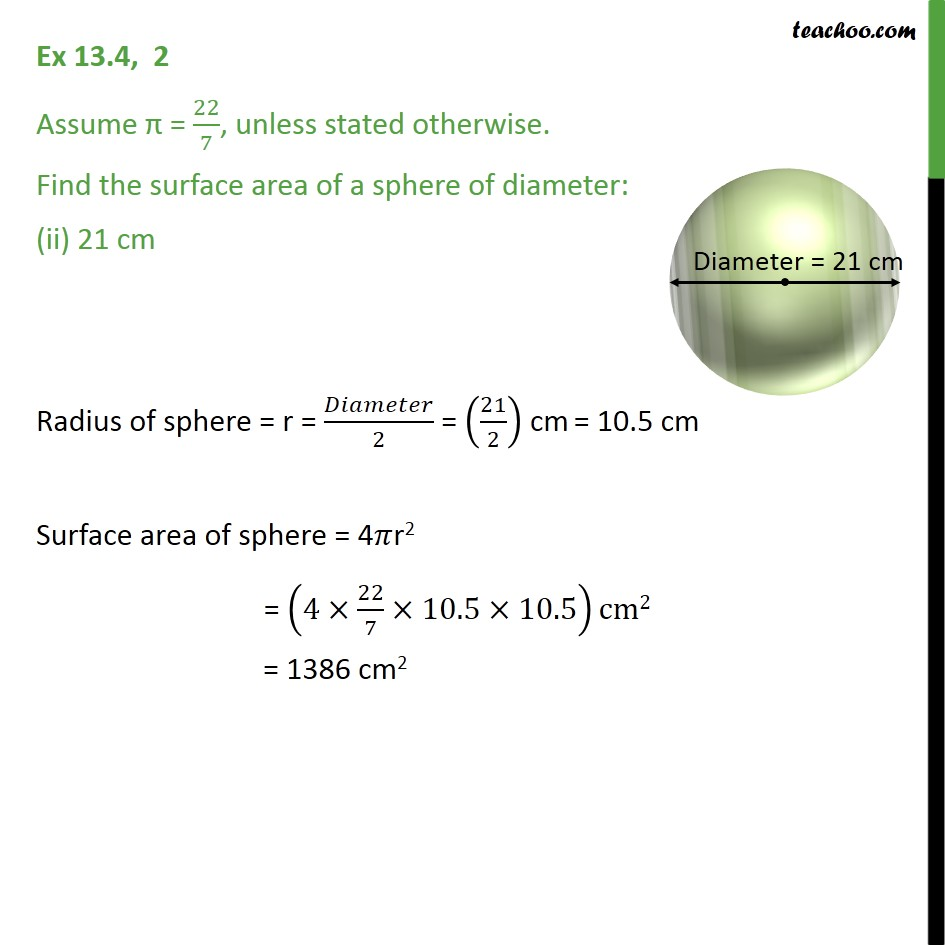Ex 11.2

Chapter 11 Class 9 Surface Areas and Volumes
Serial order wiseLearn in your speed, with individual attention - Teachoo Maths 1-on-1 Class

### Transcript

Ex 11.2, 2 Assume = 22/7, unless stated otherwise. Find the surface area of a sphere of diameter: (ii) 21 cm Radius of sphere = r = /2 = (21/2) cm = 10.5 cm Surface area of sphere = 4 r2 = (4 22/7 10.5 10.5)cm2 = 1386 cm2